Actions

An approximation of sample quantiles by empirical distribution functions.

Let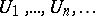be a sequence of independent uniform-random variables (cf. also Random variable). Writefor the empirical distribution function (cf. Distribution function; Empirical distribution) of the firstrandom variables and denote the uniform empirical process by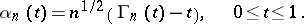Let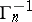be the left-continuous inverse or quantile function (cf. also Quantile) corresponding toand write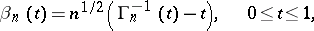for the uniform quantile process. Denote the supremum norm on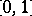by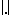. It is easy to show thata.s., implying, e.g., that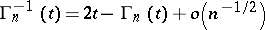a.s.,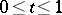. The processwas introduced by R.R. Bahadur in [a3] and further investigated by J.C. Kiefer in [a11], [a12]. Therefore this process is called the (uniform) Bahadur–Kiefer process. A final and much more delicate result foris(a1)

see [a7], [a8], [a12], [a13]. From the well-known results for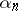it now immediately follows from (a1), that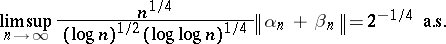(a2)

and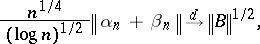whereis a standard Brownian bridge (cf. Non-parametric methods in statistics). Similar results exist for a single, fixed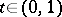:where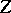is standard normal (cf. Normal distribution) and independent of. Extensions of the latter two results to finitely many's also exist, see [a4], [a5].

Letbe a continuous distribution function on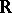, with quantile function, and set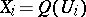,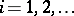. Then theare independent and distributed according to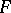. Now define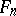to be the empirical distribution function of the firstof theand write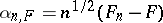for the corresponding empirical process. Denote the empirical quantile function byand define the quantile process by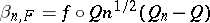, where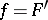. The general Bahadur–Kiefer process is now defined as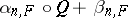. Since, results forcan be obtained when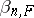is "close" to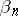. Under natural conditions, see e.g. [a13], results hold which imply that for any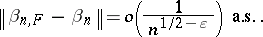This yields all the above results withreplaced with. Observe that (a2) now leads to the following Bahadur representation: Ifis bounded away from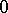, then uniformly in,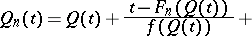There are many extensions of the above results, e.g., to various generalizations of quantiles (one- and multi-dimensional) [a1], [a9], to weighted processes [a4], [a7], to single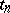's converging to[a6], to the two-sample case, to censorship models [a5], to partial-sum processes [a7], to dependent random variables [a2], [a4], [a10], and to regression models [a9].